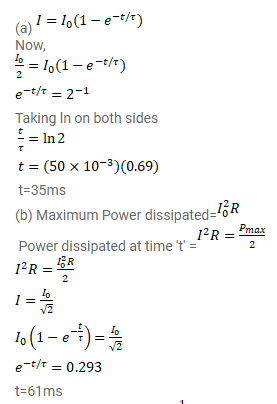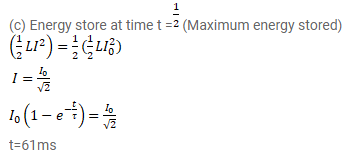# An LR circuit having a time constant of

Question:

An LR circuit having a time constant of $50 \mathrm{~ms}$ is connected with an ideal battery of emf $\varepsilon$. Find the time elapsed before

(a) the current reaches half its maximum value,

(b) the power dissipated in heat reaches half its maximum value and

(c) the magnetic field energy stored in the circuit reaches half its maximum value.

Solution: